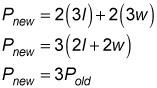##### GED Test: 1,001 Practice Questions For DummiesIf the GED Math test gives you a question involving the perimeter or area of a rectangle, you'll save time if you memorize those formulas beforehand. The following practice questions will let you know if you need to refresh your memory.

## Practice Questions

Both questions are based on the following rectangle.1. What is the area of the rectangle? (Give your answer to the nearest square inch.) A. 10 B. 17 C. 18 D. 210
2. If the rectangle were enlarged by a factor of 3 (so that its sides became 3 times as long as they were previously), what would be the ratio of the new perimeter to the old perimeter? A. 1:3 B. 1:9 C. 3:1 D. 9:1

1. The correct answer is D.

The width of the rectangle is given as 7 inches. Note that the length of the rectangle is 2.5 feet, which needs to be multiplied by 12 to convert into inches:

l = 2.5 (12) = 30

Multiply the length by the width to calculate the area of the rectangle:

A = lw = (30)(7) = 210

Hence, Choice (D) is the correct answer.

2. The correct answer is C.

The perimeter P of a rectangle of length l and width w is given by the following formula:

P = 2l + 2w

The new length of the rectangle is 3l and the new width is 3w, so the new perimeter will simply be 3 times longer than the old perimeter:Hence, the ratio of the new perimeter to the old perimeter is 3:1, making Choice (C) correct.